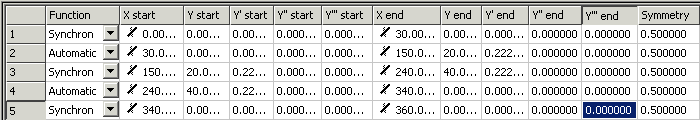# Tables Window

The values for the motion section are displayed in the table window:Description

Function

Indicates the function type (see function types).

X start

Initial value of the master position.

(The icon in front of the value indicates the type of the point.)

Y start

Initial value of the slave position.

Y' start

Initial value of the slave velocity.

Y'' start

Initial value of the slave acceleration.

Y''' start

Start value of the slave jerk.

X end

End value of the master position.

(The icon in front of the value indicates the type of the point.)

Y end

End value of the slave position.

Y' end

End value of the slave velocity.

Y'' end

End value of the slave acceleration.

Y''' end

End value of the slave jerk.

Symmetry

Symmetry value of the law of motion.

You can change the values using the keyboard.
The restrictions imposed by the choice of the function type or the boundary conditions for the points are taken into account.

Since motion sections are normally contiguous - except for Slide Points - the end point and its derivatives at the end of the section is equal to the corresponding values at the start of the following motion section.

Therefore, you should normally always manipulate the initial values.

In addition, if you find any discrepancies in a finished motion diagram, check that the start and end points match.

If certain values in the table cannot be changed, you should reconsider the boundary conditions of the points and change them if necessary.
The boundary conditions limit the scope of the functions in sections in accordance with their type.

The symmetry of the functions can only be changed for the following types:

• Polynomial3,
• Polynomial5,
• Polynomial8,
• Sineline,
• ModSineline,
• Bestehorn,
• AccTrapezoid.

Normally the inflection on the curve (acceleration = 0) at 50 % = 0.5.

This value can be modified not only in the table, but also in the graph (see Example 6).

## Function Types

In addition to the standard types (synchronous/automatic), which can be changed by command on the graph, the function type can also be modified in the combobox. When the combobox - or a field in the first column - is first clicked, a rectangle is temporarily shown in the position window, with the start and end points of the section at its corners. As soon as another field in the table window is tapped, either the rectangle for this field is shown or no rectangle.

The types correspond to those of VDI Guideline 2143. In addition, the cubic splines with the boundary conditions natural, tangential and periodic are added.

Type

Description

Boundary condition

Synchronous

Synchronous motion (constant transmission ratio between slave and master, corresponds to normalized velocity).

Constant velocity v, acceleration a=0

Automatic

Automatic adaptation to the boundary values (velocity, acceleration).

Polynomial3

3rd order polynomial

v=0, a=0

Polynomial5

5th order polynomial (restricted version rest in rest)

v=0, a=0

Polynomial8

8th order polynomial

v=0, a=0

Sineline

Sineline (see VDI Guideline 2143)

v=0, a=0

ModSineline

Modified Sineline (see VDI Guideline 2143)

v=0, a=0

Bestehorn

Bestehorn Sineline (see VDI Guideline 2143)

v=0, a=0

AccTrapezoid

Acceleration trapezoid

v=0, a=0

SinusSyncKombi

Sineline combination

v=0, a=0

ModSineline_VV

Modified Sineline for velocity in velocity.

a=0

HarmonicKombi_RT

Harmonic combination of rest in turn.

v=0; start point: a=0

HarmonicKombi_TR

Harmonic combination of rest in turn.

v=0; end point: a=0

HarmonicKombi_VT

Harmonic combination of velocity in turn.

Start point: a=0; end point: v=0

HarmonicKombi_VT

Harmonic combination of turn in velocity.

Start point: v=0; end point: a=0

AccTrapezoid_RT

Acceleration trapezoid for rest in turn.

v=0; start point: a=0

AccTrapezoid_RT

Acceleration trapezoid for turn in rest.

v=0; end point: a=0

Polynomial7_MM

7th order polynomial with adaptation to the boundary values (velocity, acceleration and jerk).

Spline

Internal section of a cubic spline.

Spline Natural

Start or end section of a natural cubic spline.

a=0

Spline Tangential

Start or end section of a tangential cubic spline.

Spline Periodic

Start or end section of a cyclic cubic spline.

Polyline

Start or end section of a linear spline.

Changing the type of spline at the first point implies that the spline type as a whole is changed, including that of the end point.

If you select the Spline Tangential spline type, you should modify the boundary conditions (first derivative at the start point and end point).

For the laws of motion with boundary conditions, R stands for rest, V for velocity, T for turn and M for Motion.